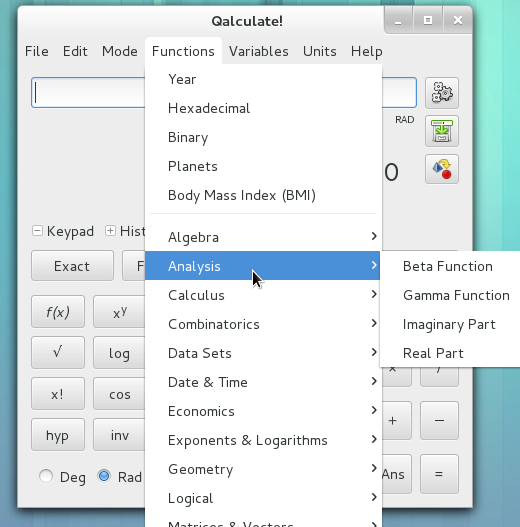# Tip of the week 10

## Qalculate - the ultimate desktop calculator !

According to the official website, Qalculate is : a multi-purpose desktop calculator for GNU/Linux. It is small and simple to use but with much power and versatility underneath. Features include customizable functions, units, arbitrary precision, plotting, and a user-friendly interface (KDE or GTK+).

Qalculate is also coming with a command line tool called qalc

## Installation

Qalculate is available on our favorite distro defaults repos (debian, ubuntu, fedora...), so you just have to use your package installer, on Fedora :

```natjohan# yum install qalculate
```

Note : There is a GUI version : qalculate-gtk or qalculate-kde

```natjohan# yum install qalculate-gtk
```

You should read the official documentation for this GUIFor your first use of qalc command :

```natjohan\$ qalc
You need the download exchange rates to be able to convert between different currencies.
You can later get current exchange rates with the "exchange rates" command.
Do you want to fetch exchange rates now from the Internet (default yes)?
> exit
```

## Usage

Here is few examples of qalc command, unfortunately there isn't man page but the synthax is easy to understand and use.

### Currency converter

So now your able to convert currencies from your command line, example :

```natjohan\$ qalc 1EUR = xCAD
(1 * euro) = (x * CAD) = x = 1.3458
natjohan\$ qalc 1EUR = xCHF
(1 * euro) = (x * CHF) = x = 1.2272
natjohan\$ qalc 12EUR + 155USD = x GBP
((12 * euro) + (155 * dollar)) = (x * GBP) = - x = 111.67764
```

Many currencies are available, just type the iso 4217 code

### Unit converter

Works with many units :

```natjohan\$ qalc 11km/52min = x km/h
((11 * kilometer) / (52 * minute)) = ((x * kilometer) / hour) = - x = 12.692308
natjohan\$  qalc 1800cal = x J
(1800 * calorie) = (x * joule) = x = 7536.24
natjohan\$  qalc 40miles = x km
(40 * mile) = (x * kilometer) = x = 64.37376
natjohan\$ qalc 40nautical_miles = x km
(40 * nautical_mile) = (x * kilometer) = x = 74.08
natjohan\$ qalc 65l / 877km = x l/100km
((65 * liter) / (877 * kilometer)) = ((x * liter) / (100 * kilometer)) = - x = 7.4116306
natjohan\$ qalc 1 years = x second
(1 * year) = (x * second) = x = 31557600
```

To calculate Unix timestamp :

```natjohan\$ qalc timestamp 2013-02-25
timestamp("2013-02-25") = 1.3617468E9
```

To solve problems like : "How many days will it take to transfer 200GiB at 1.25MiB/sec" :

```natjohan\$ qalc "200Gibyte / 1.25(Mibyte/second) = x day"
((200 * gibibyte) / (1.25 * (mebibyte / second))) = (x * day) = - x = 1.8962963
```

### Functions & variables

Qalculate comes with many functions : Economic, Statistics, geometry, etc and variables like physical constants. That a great point for scientists :P

### Interactive mode

Note you can use previous example in interactive mode, simply launch qalc command

```natjohan\$ qalc
>
```

### Tips

To find function or unit name you can launch the GUI and made an insert, or if you don't have the GUI : just go in /usr/share/qalculate and cat the xml files.

Category: ToTW
Tags: tip linux calculate qalculate qalc